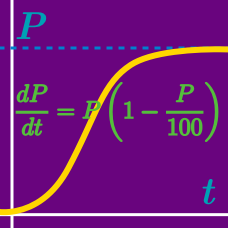Calculus

# Differential Equations - Euler's Method - Step size of 1

Consider a function $f(x)$ such that $f(1) = 4$ and $f'(x) = x^3$. Using Euler's method with step size $1,$ what is the resulting approximation of $f(9)-f(5)?$

Consider a function $f(x)$ satisfying $f(1) = 4$ and $f'(x) = x^2$. Using Euler's method with step size $1,$ what is the resulting approximation of $f(6)$?

Consider a function $f(x)$ satisfying $f(1) = 3$ and $f'(x) = \frac{1}{x(x+1)}.$ Using Euler's method with step size $1,$ what is the resulting approximation of $f(10)$?

Consider a function $f(x)$ such that $f(1) = 5, f'(x) = 4x^2 - x$. Using Euler's method with step size $1,$ what is the value of $a$ that gives the approximation $f(a) \approx 348$?

Consider a function $f(x)$ such that $f(1) = 10$ and $f'(x) = x^2 + x$. Using Euler's method with step size $1,$ what is the resulting approximation of $f(6) + f(12)$?

×